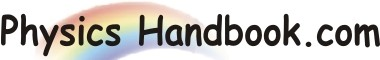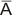HOME TOPICS DEFINITIONS TABLES LAWS INVENTIONS EXPERIMENTS QUIZ VIDEOS
 A B C D E F G H I J K L M N O P Q R S T U V W X Y Z
Boolean Algebra
Digital circuits perform the binary arithmetic operations with binary digits 1 and 0.These operations are called logic function or logical operations. The algebra used to symbolically describe logic function is called Boolean Algebra.
Boolean Algebra is a set of rules and theorems mathematically. As with the ordinary algebra, the letters of alphabet (e.g. A, B, C etc.) can be used to represent the variables. Boolean Algebra differs from ordinary algebra in that Boolean constant and variable can have only two values; 0 and 1. There are four connecting symbols used in Boolean Algebra viz.
(I) Equals sign (=)
(II) Plus sign (+)
(III) Multiply sign (.)
(IV) Bar(-)
(I) Equals sign (=): The equals sign in Boolean Algebra refers to standard mathematical equality. In other words, the logical value on one side of the sign is identical value on the other side of the sign. Suppose we are given two logical variables such that A = B. Then if A = 1 ,then B = 1 and if A = 0 then B = 0.
(II) plus sign (+): The plus sign in Boolean Algebra refers to logical OR operation. Thus, when the statement A + B = 1 appears in Boolean Algebra, It means A OR with B equals 1. Consequently, either A = 1 or B = 1 or both equal 1.
(III) Multiply sign (.): The multiply sign in Boolean Algebra refers to logical AND operation. Thus, when the statement A . B = 1 appears in Boolean Algebra, It means A AND with B equals 1. Consequently, either A = 1 and B = 1.The Function A . B is often written as AB, omitting the dot for convenience.
(IV) Bar sign (-): The bar sign in Boolean Algebra refers to logical NOT operation. The NOT has the effect of inverting (complementing) the logical value. Thus, if A = 1 then= 0 .